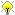[Documentation] [TitleIndex] [WordIndex]

 Note: This tutorial assumes that you have completed the previous tutorials: basic usage of roslisp.Please ask about problems and questions regarding this tutorial on answers.ros.org. Don't forget to include in your question the link to this page, the versions of your OS & ROS, and also add appropriate tags.

# Basic usage of CL_TF

Description: This tutorial describes the tf client that allows easy access to transformations in the ROS TF framework

Tutorial Level: INTERMEDIATE

### Working with TF

TF is the transformation framework with which we can transform between different coordinate systems distributed over independent modules at runtime in ROS. CL_TF is a ros package that defines a suitable common lisp library to do so.

CL_TF uses the library cl_transforms, which contains the geometric functions used. CL_TF adds the ROS TF publishing and subscribing of poses and frames.

Using TF, if we want the arm to point at an object perceived by a camera, or the camera to look at the hand of the robot, the functionality to transform from one frame to the other needs not be implemented twice for each controller. Instead, both controllers publish their transforms to ROS, and use published transforms to do calculations.

We can load the definitions into the REPL using:

`CL-USER> (ros-load:load-system "cl_tf" "cl-tf")`

or work in a package having this dependency declared.

One type that you will use a lot with TF is the stamped pose. For the tutorial, we switch into the CL-TF LISP package in the REPL:

`CL-USER> (in-package cl-tf)`

You will usually get this from a topic or service, but for now we create one manually:

```TF> (defparameter *front-pose*
(cl-tf:make-pose-stamped
(cl-transforms:make-3d-vector 0.5 0 1)
(cl-transforms:make-quaternion 0 0 0 1)))```

For a PR2, this indicates a pose half a meter in front of the robot and 1 meter high, as "/base_link" denotes the robot base frame. There are other functions defined like (cl-tf:make-point-stamped) for points. We use the cl-transforms library here to create a vector and quaternion.

To get the properties of any pose that you get from tf, you can use simple accessor functions:

```(tf:frame-id *front-pose*)
(tf:stamp *front-pose*)
(cl-transforms:origin *front-pose*)
(cl-transforms:orientation *front-pose*)```

We can transform the pose above into other frames, remember you can get the list of current frames and transformations using

`\$ rosrun tf tf_monitor`

To transform from one frame into another, we need a transformer. A transformer listens to the ros topic where all TF transforms publish their own properties. We can instantiate a transformer using:

`TF> (defparameter *transform-listener* (make-instance 'cl-tf:transform-listener))`

This starts listening on the topic, and it might take a few instants until it learned about all transforms.

Now as an example, we can transform the pose above to the frame of the stereo cameras:

```TF> (cl-tf:transform-pose *transform-listener* :pose *front-pose* :target-frame "/wide_stereo_optical_frame")

#<CL-TF:POSE-STAMPED
#<3D-VECTOR (0.030038491171086697d0, -0.09739889466661444d0, 0.49539886544002415d0)>
#<QUATERNION v: (-0.6565981279699529d0, 0.6565535613867549d0, -0.26249472830731163d0), w: -0.26251254634320326d0>>```

Note that the actual result of course depends on where the robot head is pointed at.

2019-11-30 12:34